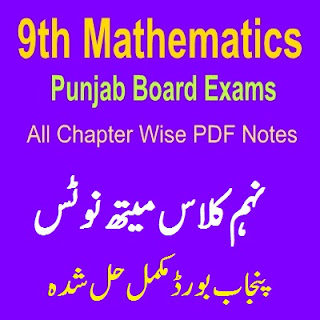# 9th Class Mathematics Notes Punjab Board

All Solved Chapter Wise Questions of 9th Class Mathematics notes for the students of Grade Nine. Fully Solved Questions with Short Questions and online MCQs Quiz tests for the students help. If you will try these notes and prepared your tests or papers then you will be able to get good marks in the final exams.9th Class Full Chapters Notes

All Chapter Wise 9th Class Notes:

Here is the list of 9th Class all Punjab Board of Pakistan Chapter Wise solved Mathematics Chapter Wise Exercises with definitions. All Definitions of the chapters are fully explained. Easily prepared the questions of solved Mathematics Guide.

Easily prepared all of these Chapters that list is below explained with 9th class mathematics chapter name also defined after the chapter numbers here.

Chapter 1 Matrices

Chapter 2 Real and Complex Numbers

Chapter 3 Logarithms

Chapter 4 Algebraic Expressions and Algebraic Formulas

Chapter 5 Factorization

Chapter 6 Algebraic Manipulations

Chapter 7 Linear Equations and Inequalities

Chapter 8 Linear Graphs and Their Application

Chapter 9 Introduced To Co-Ordinate Geometry

Chapter 10 Congruent Triangles

Chapter 11 Perallelograms and Triangles

Chapter 12 Line Bisector and Angle Bisector

Chapter 13 Sides and Angles Of A Triangles

Chapter 14 Ratio and Proportional

Chapter 15 Pythagoras Theorem

Chapter 16 Theorems Related With Area

Chapter 17 Practical Geometry

From 5th Class to 12th Class all Chapter wise fully Solved notes for the students. You have to need also try online Quiz Tests for the best Objective type MCQs tests Preparations Here.

Many of the students want to know that what is the exercise solutions of the questions. But in this PDF guides all of the 17 chapters complete exercises are explained with every question solutions.

Every question have explained in this PDF guides you can also easily printout these important notes of 9th class chapter wise explanations.

All types of short questions are also explained in the definitions of the chapter wise notes in these PDF notes of all Punjab Board of BISE 9th Mathematics.

These PDF notes are also very helpful for the Educators Preparations with the definitions point of views for Mathematics teaching jobs and others important tests of Mathematics.

All PPSC, NTS, CSS, OTS exams and Educators tests this guide is helpful for learning and solving mathematics questions with definitions explanations.

Previous Post
Next Post